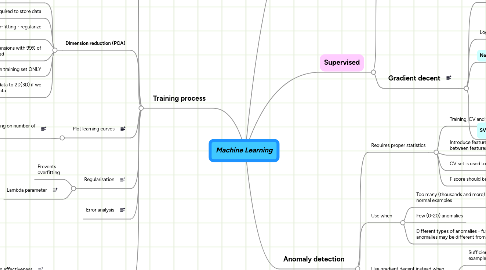# Machine Learning

Just an initial demo map, so that you don't start with an empty map list ...

Get Started. It's FreeMachine Learning## 1. Training process

### 1.1. Hints

1.1.1. Implement quickest approach first

1.1.2. More data rules

1.1.3. Can human classify?

### 1.3. Training examples

1.3.1. 60% training data

1.3.2. 20% validation data

1.3.3. 20% test data

### 1.4. Dimension reduction (PCA)

1.4.1. Use when algorithm is slow on full dataset

1.4.2. Use to decrease size required to store data

1.4.3. NOT use to fight over-fitting - regularize instead

1.4.4. Pick smalest dimensions with 99% of variance is retained

1.4.5. Calculate on training set ONLY

1.4.6. Hint: Reduce data to 2D(3D) if we need to plot data

### 1.5. Plot learning curves

1.5.1. Plot error graph depending on number of examples

1.5.1.1. High error on both training and validation sets even on large training set means high bias

1.5.1.1.1. Increase degree of polynom

1.5.1.2. If error on validation set starts increasing while error on training set is very low and decreasing then this is high variance - overfitting

1.5.1.2.1. Tune lamda coefficient in regularisation

### 1.6. Regularisation

1.6.1. Prevents overfitting

1.6.2. Lambda parameter

### 1.8. Classification error analysis

1.8.1. Classification effectiveness

1.8.1.1. F score

1.8.1.2. Precision

1.8.1.3. Recall

1.8.1.4. Metrics

1.8.1.5. Accuracy

1.8.2. Play with threshold

## 2. Supervised

### 2.1. Normal equations

2.1.1. Does not need normalisation of features

2.1.2. Requires maxtrix inversion which is slow

2.2.1. Linear regression

2.2.1.1. Number of features larger then number of examples

2.2.1.2. Small number of features large number of examples

2.2.1.3. Initialization of theta matrix may be zeros

2.2.2. Logistic regression

2.2.2.1. Initialization of theta matrix may be zeros

2.2.3. Neural network

2.2.3.1. Work for all cases but slow to train

2.2.3.2. Initialization of theta matrix must be random

2.2.4. SVM - support vector machine

2.2.4.1. Feature scaling is a must (normalization)

2.2.4.2. C parameter

2.2.4.3. Linear kernel = no kernel

2.2.4.3.1. Number of features larger then number of examples

2.2.4.3.2. Small number of features large number of examples

2.2.4.4. Gussian kernel

2.2.4.4.1. Sigma

2.2.4.4.2. Number of examples in ~times larger then number of featues

## 3. Unsupervised

### 3.1. Clustering

3.1.1. K-Means

3.1.1.1. Algorithm

3.1.1.1.1. Select K - desired number of culsters

3.1.1.1.2. Select K centroids

3.1.1.1.3. Split examples by clusters based on distance to centroids

3.1.1.1.4. Recalculate cluster centroid - average of examples in it

3.1.1.1.5. Repeat until cost function is decreasing

3.1.1.2. Hints

3.1.1.2.1. If K ~ 2-10 then algorithm should be executed 50-1000 times to avoid local optima and find lowest possible cost function value

3.1.1.2.2. If K ~ 100 there is no sense to run algorithm several times - because first result will be very close to global optima on most of training sets sizes

## 4. Anomaly detection

### 4.1. Requires proper statistics

4.1.1. Training, CV and test sets are used

4.1.1.1. Training set should not have anomalies

4.1.1.2. CV and test sets shoud have anuomalies

4.1.2. Introduce features to catch correlations between features

4.1.3. CV set is used to choose epsilon parameter

4.1.4. F score should be used to evaluate efficiency

### 4.2. Use when

4.2.1. Too many (thousands and more) normal examples

4.2.2. Few (0-20) anomalies

4.2.3. Different types of anomalies - future anomalies may be different from examples

4.3.1. Sufficient number of positive and negative examples

4.3.2. Anomalies have similarties and may and future will be similar to registered (spam for example)

### 4.4. Basic model

4.4.1. Additional features to catch correlations between featues should be introduced manually

4.4.2. Computational cheap - may work on 10k-100k features

4.4.3. May work even if training set is small

### 4.5. Multivariate model

4.5.1. Automatically catch correlations between features

4.5.2. Computaionally more expansive

4.5.3. Must have more examples in training set then features